Copyright © Michael Richmond. This work is licensed under a Creative Commons License.

# Estimating Distances across our Galaxy

Hipparcos measurements provide accurate measurements of distance for stars which are within about 100 to 200 parsecs. That includes a lot of stars, but is only a small fraction of the size of our galaxy. How can we extend these results for nearby stars to objects halfway across our Milky Way Galaxy, or to stars in other galaxies?

Part one of the answer is to use the inverse square law to calculate the distance to an object, given its apparent brightness -- which is easy to measure -- and its intrinsic luminosity. But how to we know a star's intrinsic luminosity?

Well, we can start by making some very simple (and wrong) assumptions, and then try to improve those assumptions.

• all stars have the same luminosity (simple and wrong)
• all stars have the same relationship between color and luminosity (less simple, less wrong)
• all main sequence stars have the same relationship between color and luminosity (complicated, but correct enough for most purposes)

#### The Inverse Square Law

Stars behave like all light-emitting bodies: their apparent brightness decreases if one moves them farther away. In fact, there is a simple mathematical rule which describes the change in a star's apparent brightness as a function of its distance away from us:

```                                                          2
apparent brightness at distance A       [  distance B  ]
---------------------------------   =   [ ------------ ]
apparent brightness at distance B       [  distance A  ]
```
This is called the inverse square law, because the ratio of brightnesses depends on the square of the inverse of the ratio of distances.

For example, suppose a star is exactly 10 parsecs away from us. We can measure its brightness -- call it "B". Now, if an alien race moves the star so that is becomes 20 parsecs away from us, we will measure a brightness which is only one-fourth of "B".

Another way to write the inverse square law involves the luminosity of a star. Luminosity is the total amount of energy emitted by a star each second; it can be measured in Watts, just like a light bulb. The luminosity is an intrinsic property of a star -- it doesn't depend on the star's distance from us in any way. The apparent brightness of a star can be written as

```                                        luminosity of star
apparent brightness of star   =   ----------------------
4 * pi * (distance)^2
```

Once again, note how the apparent brightness depends on the square of the distance.

So, if we know the intrinsic luminosity of a star, and we can measure its apparent brightness, then we can calculate its distance.

But how can we determine a star's intrinsic luminosity?

Two factors control the luminosity of a star:

• temperature: high temperature means more energy emitted
• size: large size means more energy emitted
Temperature turns out to be more important -- even a small change in temperature can make a very large difference in luminosity. Physicists have figured out the exact equation:
```  luminosity =   (area)     *   (energy emitted per area)

2                   4
= ( 4 pi R  )  *   ( sigma * T  )
```
where
• R is the radius of the star
• T is the temperature of the star, on the Kelvin scale
• sigma is the Stefan-Boltzmann constant; you can look up its value in a book.

So, if two stars are the size size, but different temperatures, the hotter one is more luminous: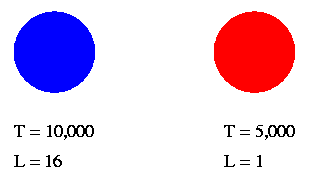The difference grows very rapidly with temperature:

```   ratio of temperature          ratio of luminosity
----------------------       ------------------------
1                              1
1.1                            1.5
1.5                            5
2                             16
3                             81
10                         10,000
```

If two stars are the same temperature, but different sizes, then the larger one is more luminous: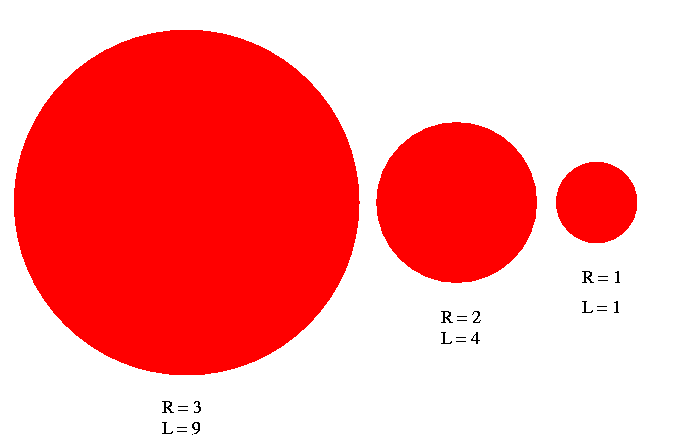The difference grows more slowly with size:

```   ratio of radius               ratio of luminosity
----------------------       ------------------------
1                              1
1.1                            1.2
1.5                            2.3
2                              4
3                              9
10                            100
```

The bottom line is that, unless we know both the temperature and the size of a star, we can't figure out its luminosity ... and that will prevent us from using its apparent brightness to figure out its distance. Rats.

#### The color-magnitude diagram

We want to figure out the temperature and size of a star, so that we can determine its distance.

Temperature is actually pretty easy to determine:

• the color of a star gives a decent estimate of its temperature: blue stars are hot, red stars are cool. Color is very easy to measure; just compare the amount of light seen through a blueish filter with the amount seen through a reddish filter.

• the spectrum of a star provides a much more accurate estimate of its temperature. The relative darkness of absorption lines of different elements can be compared to atomic properties, and models of the stellar atmosphere.

The real problem is figuring out the size of a star. Hmmmm...

But, in an exception to the general rule, the universe turns out to be nice to astronomers (for once): there is a fundamental relationship between the temperature of a star and its size, which is true for about 90% of all stars. This relationship is revealed in the color-magnitude diagram, which is also called the Hertzsprung-Russell diagram.

Hipparcos measured the colors (and hence rough temperature) for lots of nearby stars. It also measured the distance to those stars, from which we can derive their luminosities. If we plot the luminosity versus the color, we find this: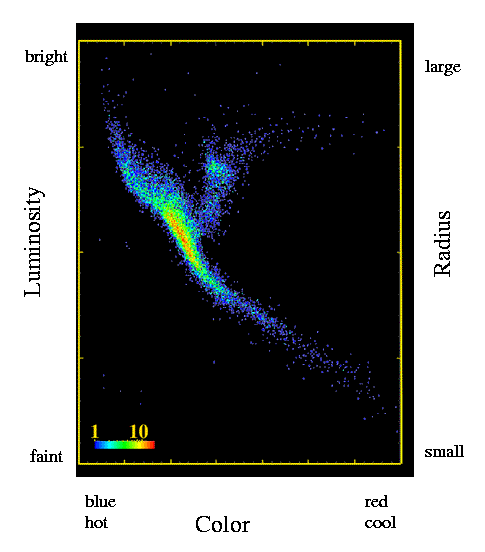Rather than being scattered all over the graph, almost all the stars fall into a band which runs from upper-left to lower-right. This band is called the main sequence. Stars along this band

• are burning hydrogen into helium in their cores
• vary in temperature, from about 40,000 at the blue end to about 3,000 at the red end
• vary in size -- the biggest blue stars are about 10 times larger than the Sun, and the smallest red are about 10 times smaller than the Sun.
• are called "dwarfs" (yes, even though they do range quite a bit in size)

It turns out that the relatively simple relationship between temperature and size stems from the fact that all these stars are powered by the same fusion reactions in their cores.

Anyway, one important consequence is that we can almost use the color of a star (easy to measure) to guess its luminosity ... and that would give us its distance.

• measure color of star through several filters
• use color-magnitude diagram to turn color into luminosity
• use luminosity and apparent brightness to get distance

But -- there's one problem with this method: the giants.

#### Dwarfs versus Giants

A small fraction of stars (perhaps 5 percent) do not obey the ordinary relationship between color and size. These stars, called giants, are much, much larger than ordinary stars of the same temperature. Why? Because giant stars are not simply fusing hydrogen to helium in their cores; some have shells of fusing material surrounding an inert core, others are fusing helium into heavier elements. The differences in energy generation eventually (through a long chain of physical phenomena) cause the outer layers of these stars to move much farther away from the cores.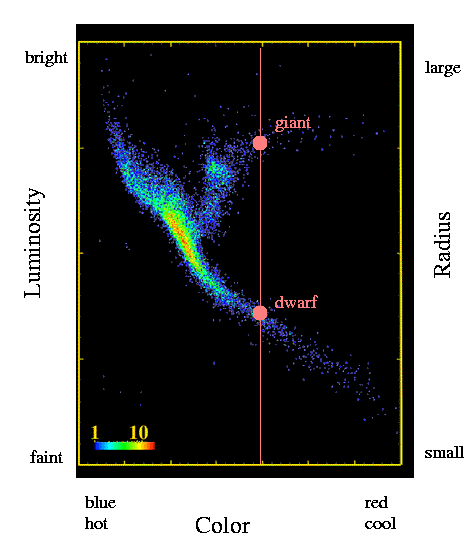If we look at one particular star, and measure its color and apparent brightness, there are sometimes two possible values for its luminosity:

• a relatively small luminosity, if it lies on the main sequence; this would mean it is relatively close to us ...
• a much higher luminosity, if it happens to be a giant star; this would mean that it is relatively far away ...

Now, most of the time, the star would turn out to be on the main sequence, so we could just always make that assumption. But even a small fraction of mis-classified stars with very wrong distances could screw up our whole view of the Galaxy.

Is there any way to separate the dwarfs from the giants, so that we can combine the apparent brightness of a star and its inferred luminosity to calculate its distance safely?

#### Using clusters to distinguish dwarfs and giants

Yes! One solution is to concentrate on clusters of stars. There are several types of stellar clusters:

Open clusters contain mostly young stars, with lots of hot, blue stars: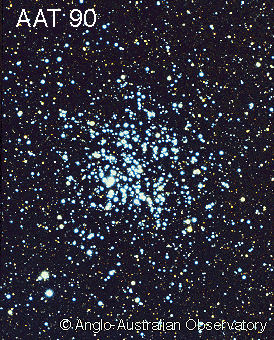Globular clusters have many more stars, most of which are relatively old, cool, and red: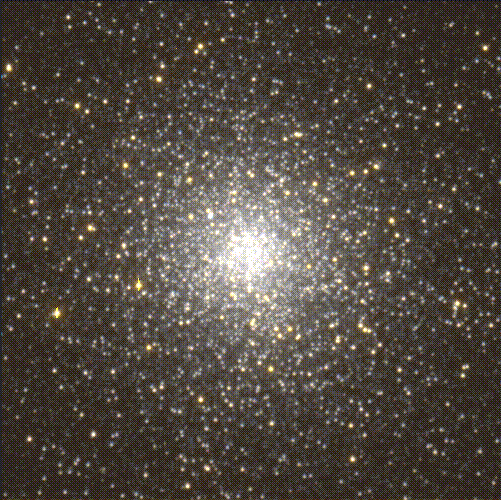The important thing about clusters is that all the stars in the cluster are at (nearly) the same distance from Earth. This means that if we make a color-magnitude diagram for stars which belong to a cluster, the difference between main-sequence stars and giants is easy to see: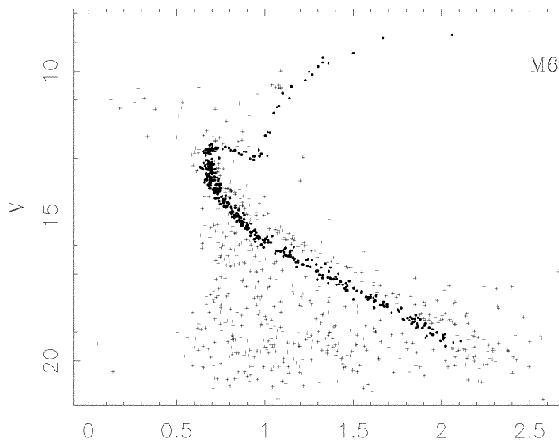This diagram, for the open cluster called M67, shows that if we pick a star with color = 1, then

• if it has a brightness of about magnitude V = 16 , it's on the main sequence
• if it has brightness of about magnitude V = 13, it's a giant

Suppose we look at another open cluster -- for example, the one called NGC 188: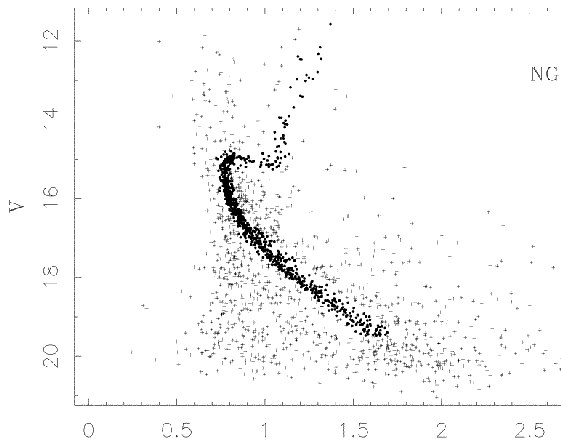In this case, if we look at a star with color = 1, then

• if it has a brightness of about magnitude V = 17.5 , it's on the main sequence
• if it has brightness of about magnitude V = 15, it's a giant

Great! Now, we can make a fair comparison of the distance between the two clusters, simply by matching up the stars on the main sequence with the same colors. It looks like we need to slide a star at color = 1 in NGC 188 upwards by about 1.5 magnitudes to make it match the brightness of a similar star in M67. That means that M67 is closer than NGC 188; and, using the inverse square law, we can figure out exactly how much closer.

So, simply by comparing the color-magnitude diagrams of two star clusters, we can figure out their relative distances from Earth. But how can we determine their absolute distances?

Simple -- thanks to Hipparcos. The Hipparcos satellite provided us with information with which we can make a color-magnitude diagram for stars with KNOWN distances. So, we just compare the color-magnitude diagram for a star cluster to the color-magnitude diagram from Hipparcos;

• the Hipparcos diagram gives us the luminosity for stars
• the cluster diagram gives us the apparent brightness for stars
Using the inverse square law, we can calculate the actual distances to the stars. Rah, rah!Copyright © Michael Richmond. This work is licensed under a Creative Commons License.